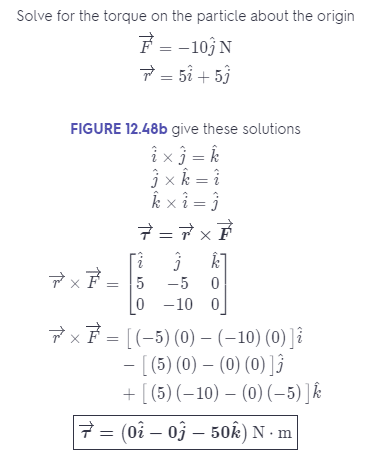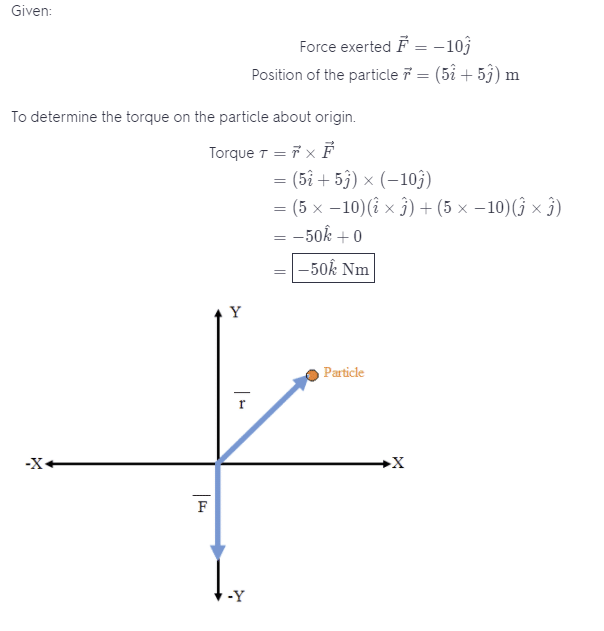# What Is The Torque On The Particle About The Origin?

We thoroughly check each answer to a question to provide you with the most correct answers. Found a mistake? Let us know about it through the REPORT button at the bottom of the page.

Force F = -10jN is exerted on a particle at r = (5i + 5j) m. What is the torque on the particle about the origin?

Answer: r = (-50k) N * m.

## Explanation